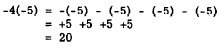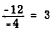Custom SearchMULTIPLICATION To explain the rules for multiplication of signed numbers, we recall that multiplication of whole numbers may be thought of as shortened addition. Two types of multiplication problems must be examined; the first type involves number8 with unlike signs, and the second involves numbers with like signs. Unlike Signs Consider the example 3(-4), in which the multiplicand is negative. This means we are to add -4 three times; that is, 3(-4) is equal to (-4) + (-4) + (-4), which is equal to -12. For example, if we have three 4-dollar debts, we owe 12 dollars in all. When the multiplier is negative, as in -3(7), we are to take away 7 three times. Thus, -3(7) is equal to -(7) - (7) - (7) which is equal to -21. For example, if 7 shells were expended in one firing, 7 the next, and 7 the next, there would be a loss of 21 shells in all. Thus, the rule is as follows: The product of two numbers with unlike signs is negative. The law of signs for unlike signs is sometimes stated as follows: Minus times plus is minus; plus times minus is minus. Thus a problem such as 3(-4) can be reduced to the following two steps: 1. Multiply the signs and write down the sign of� the answer before working with the numbers themselves. 2. Multiply the numbers as if they were unsigned numbers. Using the suggested procedure, the sign of the answer for 3(-4) is found to be minus. The product of 3 and 4 is 12, and the final answer is -12. When there are more than two numbers to be multiplied, the signs are taken in pairs until the final sign is determined. Like Signs When both factors are positive, as in 4(5), the sign of the product is positive. We are to add +5 four times, as follows: 4(5) = 5 + 5 + 5 + 5 = 20 When both factors are negative, as in -4(-5), the sign of the product is positive. We are to take away -5 four times.Remember that taking away a negative 5 is the same as adding a positive 5. For example, suppose someone owes a man 20 dollars and pays him back (or diminishes the debt) 5 dollars at a time. He takes away a debt of 20 dollars by giving him four positive 5-dollar bills, or a total of 20 positive dollars in all. The rule developed by the foregoing example is as follows: The product of two numbers with like signs is positive.  Knowing that the product of two positive numbers or two negative numbers is positive, we can conclude that the product of any even number of negative numbers is positive. Similarly, the product of any odd number of negative numbers is negative. The laws of signs may be combined as follows: Minus times plus is minus; plus times minus is minus; minus times minus is plus; plus times plus is plus. Use of this combined rule may be illustrated as follows: 4(-2) - (-5) - (6) - (-3) = -720 Taking the signs in pairs, the understood plus on the 4 times the minus on the 2 produces a minus. This minus times the minus on the 5 produces a plus. This plus times the understood plus on the 6 produces a plus. This plus times the minus on the 3 produces a minus, so we know that the final answer is negative. The product of the numbers, disregarding their signs, is 720; therefore, the final answer is -720. Practice problems. Multiply as indicated: 1. 5(-8) = ? 2. -7(3) (2) = ? 3. 6(-1)(-4) = ? 4. -2(3)(-4)(5)(-6) = ? Answers: 1. -40  2. -42  3. 24 4. -720 DIVISION Because division is the inverse of multiplication, we can quickly develop the rules for division of signed numbers by comparison with the corresponding multiplication rules, as in the following examples: 1. Division involving two numbers with unlike signs is related to multiplication with unlike signs, as follows: Therefore,Thus, the rule for division with unlike signs is: The quotient of two numbers with unlike signs is negative. 2. Division involving two numbers with like signs is related to multiplication with like signs, as follows:             3(-4) = -12 Therefore,Thus the rule for division with like signs is: The quotient of two numbers with like signs is positive. The following examples show the application of the rules for dividing signed numbers:Practice problems. Multiply and divide as indicated:Answers: 1. -3  2. -1  3. 2 4. -9